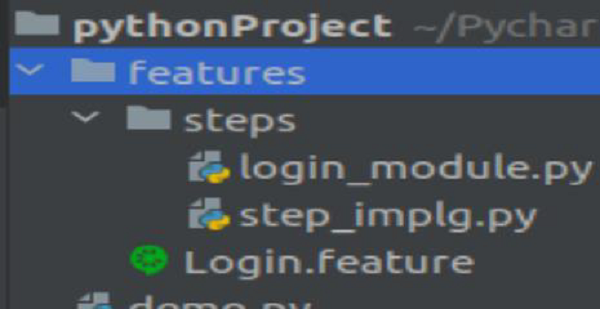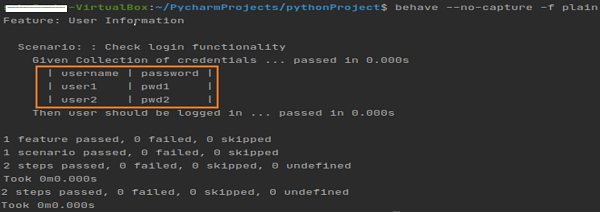# Behave - Setup Table

A step can have a text and data table associated with it. We can add a data table with a step. It is recommended to have the table data indented and it is mandatory to have an equal column number for each line.

A column data should be separated by the | symbol.

The feature file is as mentioned below −

Feature − User Information
Given Collection of credentials
| user1    | pwd1    |
| user2    | pwd2    |
Then user should be logged in


A table is accessible to the implementation Python code with the .table attribute within the context variable (passed in the step function). A table is an instance of Table. We can use the set up table to facilitate setting up the test.

Python code

The python code to access table.(login_module.py) is as follows −

class Deprt(object):
if not ms:
ms = []
self.ms = ms
assert usernane not in self.ms
self.ms.append(usernane)
class LModel(object):
def __init__(self):


Corresponding Step Implementation File(step_implg.py)

The file is as follows −

from behave import *
@given('Collection of credentials')
def step_impl(context):
model = getattr(context, "model", None)
if not model:
context.model = LModel()
#iterate rows of table
for r in context.table:
@then('user should be logged in')
def step_impl(context):
pass


Project setup

The project set up for the file in Python project is as followsOutput

The output obtained after running the feature file is given below and the command used is behave --no-capture -f plain.The output shows the step up table printed.